본문바로가기

## 검색결과

검색결과 (전체 1건)

### 검색결과제한

열기
• 소장도서관
• 원문
• 발행년도
-
• 언어
• 원문이용안내
•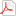모든 이용자
•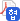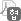협정기관 이용자
•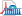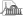국회도서관 방문 이용자
• 원문아이콘이 없는 자료는 국회도서관에 방문하여 책자로만 이용이 가능합니다.

논문명/저자명
소형 무인 헬리콥터의 모델 식별 및 강인 자세 제어기 설계 = System identification and design of a robust controller for an unmanned small scale helicopter / Hardian Reza Dharmayanda
발행사항
서울 : 건국대학교 대학원, 2010.8
청구기호
TD 629.47 -10-114
형태사항
xii, 129 p. ; 26 cm
자료실
전자자료
제어번호
KDMT1201059266
주기사항
학위논문(박사) -- 건국대학교 대학원, 항공우주정보시스템공학, 2010.8. 지도교수: 강태삼
원문
미리보기

목차보기더보기

Title Page

Contents

Nomenclature 12

Abstract 14

Chapter 1. Introduction 16

1.1. Research motivations 16

1.2. Prior work and relevant research 17

1.3. Research contribution 20

1.4. Dissertation outline 21

Chapter 2. Integration of Vehicle, Hardware and Software 22

2.1. Vehicle Platform 22

2.1.1. Raptor E620 platform 24

2.1.2. FLYUS 50EP platform 29

2.2. Onboard System 40

2.2.1. Onboard system of Raptor E620 40

2.2.2. Onboard system of FLYUS 50EP 43

2.3. System Integration 47

Chapter 3. RUAV Dynamics Modeling and System Identification 52

3.1 Coordinate Systems 53

3.1.1. Inertial reference and earth coordinate system 53

3.1.2. Body coordinate system 54

3.2. General RUAV model 55

3.2.1. Euler Newton equation 55

3.2.2. Forces and moments 56

3.2.2.1. Main Rotor and Stabilizer bar 58

3.2.2.2. Tail Rotor 60

3.2.2.3. Fuselage and fins 60

3.2.3. Linearization 61

3.2.4. State space equations 65

3.3. System Identification 66

3.3.1. Flight data acquisitions 68

3.3.2. Data consistency and reconstruction 70

3.3.3. Model structure determination 73

3.3.4. Identification Process 74

3.3.4.1. Identification algorithm 75

3.3.4.2. Identification steps 77

3.3.5. Validation and application 78

3.4. Identification of Raptor E620 79

3.5. Identification of Flyus 50EP 90

Chapter 4. Controller Design for RUAV 98

4.1. Control system introduction 98

4.2. Notation and terminology 101

4.3. H∞ formulation and solution 104

4.4. Attitude control design of hovering RUAV 107

4.4.1. Controller design for Raptor E620 110

4.4.2. Raptor's classical controller design 114

4.4.3. Flyus's H∞ Controller design(이미지참조) 117

4.5. Controller validation 118

4.5.1. Controller validation for Raptor E620 120

4.5.2. Controller validation for Flyus 50EP 126

4.6. Flyus's autonomous hovering test 127

Chapter 5. Conclusion 132

References 134

국문초록 141

Table 2-1. Parameters of thunder Tiger Raptor E620 RC model helicopter. 24

Table 2-2. Parameters of Flyus 50EP RC model helicopter. 30

Table 2-3. Dynamic behavior of swash plate and servomotors. 34

Table 2-4. Represents the nominal value for servo 1,2,3 and 4 which will be used to determine the mixing equation as described below. 37

Table 2-5. Flight data measurements of Raptor E620. 42

Table 2-6. Flight data measurements of Flyus 50EP. 47

Table 3-1. The characteristic of Raptor's state space model. 89

Table 3-2. The characteristic of Flyus' state space model. 97

Table 4-1. Proportional gain of Raptor's attitude controller. 115

Figure 2-1. General main - tail rotor configuration. 23

Figure 2-2. Thunder Tiger Raptor E620 RC model helicopter. 25

Figure 2-3. Collective motion of control input mechanism. 26

Figure 2-4. Lateral motion of control input mechanism. 26

Figure 2-5. Longitudinal motion of control input mechanism. 27

Figure 2-6. CCPM Manager. 28

Figure 2-7. FLYUS 50EP RC model helicopter. 29

Figure 2-8. CAD drawing of FLYUS 50EP RC. 30

Figure 2-9. The servos configuration for swash plate actuation. 32

Figure 2-10. Convention of RC controller. 32

Figure 2-11. PWM signals diagram for FLYUS 50EP. 33

Figure 2-12. Dynamic behavior of servomotor. 34

Figure 2-13. Servo outputs for collective input. 35

Figure 2-14. Servo outputs for lateral cyclic input. 36

Figure 2-15. Servos outputs for longitudinal cyclic input. 36

Figure 2-16. Servos outputs for pedal input. 37

Figure 2-17. Servos distributions. 38

Figure 2-18. Raptor E620 Onboard System. 41

Figure 2-19. Sensors mounted on the vehicle. 41

Figure 2-20. Raptor equipped with onboard system. 42

Figure 2-21. IMU MI-A3330X. 43

Figure 2-22. FCC Board. 44

Figure 2-23. FCC flow chart. 45

Figure 2-24. Side view of Flyus equipped with onboard system. 46

Figure 2-25. Flow diagram of communication system of Raptor E620. 48

Figure 2-26. Flow diagram for flight data acquisition of Flyus 50EP. 49

Figure 2-27. Flow diagram for autonomous control application. 50

Figure 2-28 Control process in ground control station. 51

Figure 3-1. Coordinate systemn for small scale helicopter system. 54

Figure 3-2. Longitudinal forces and moments. 57

Figure 3-3. Lateral forces and moments. 57

Figure 3-4. Longitudinal rotor flapping dynamics. 58

Figure 3-5. Lateral rotor flapping dynamics. 59

Figure 3-6. System identification flow chart. 67

Figure 3-7. Frequency sweep input 69

Figure 3-8. Doublet input. 70

Figure 3-9. Nondeterministic error. 72

Figure 3-10. Hover flight data acquisition. 80

Figure 3-11. Raptor's tail rotor input-output response. 81

Figure 3-12. Raptor's lateral input-output response. 81

Figure 3-13. Raptor's longitudinal input-output response. 82

Figure 3-14. Raptor's collective input and translational velocity response. 82

Figure 3-15. Raptor's roll rate response. 85

Figure 3-16. Raptor's roll angle response. 85

Figure 3-17. Raptor's pitch rate response. 86

Figure 3-18. Raptor's pitch angle response. 86

Figure 3-19. Raptor's yaw rate response. 87

Figure 3-20. Raptor's forward velocity response. 87

Figure 3-21. Raptor's lateral velocity response. 88

Figure 3-22. Raptor's axial velocity response. 88

Figure 3-23. Flyus helicopter in taking off and hovering position. 90

Figure 3-24. Flyus' longitudinal input-output response. 91

Figure 3-25. Flyus' lateral input-output response. 91

Figure 3-26. Flyus' tail rotor input-output response. 92

Figure 3-27. Flyus' collective input-output response. 92

Figure 3-28. Flyus' roll rate response. 94

Figure 3-29. Flyus' roll angle response. 94

Figure 3-30. Flyus' pitch rate response. 95

Figure 3-31. Flyus' pitch angle response. 95

Figure 3-32. Flyus' yaw rate response. 96

Figure 4-1. Control loop with plant uncertainty. 99

Figure 4-2. Trade-off between performance and stability. 100

Figure 4-3. The block diagram for H∞ control. 102

Figure 4-4. Feedback system with weighting functions. 106

Figure 4-5. Framework to design H∞ controller.(이미지참조) 109

Figure 4-6. Singular values plot of the controller. 112

Figure 4-7. Singular values plot of the plant and loop gain. 113

Figure 4-8. Maximum singular values of loop characteristic functions. 113

Figure 4-9. Root locus of longitudinal cyclic (δlon) to pitch rate (q) mode.(이미지참조) 115

Figure 4-10. Root locus of longitudinal cyclic (δlon) to pitch angle (θ) mode.(이미지참조) 116

Figure 4-11. Root locus of lateral cyclic (δlat) to pitch rate (p) mode.(이미지참조) 116

Figure 4-12. Root locus of lateral cyclic (δlat) to pitch angle (Ø) mode.(이미지참조) 117

Figure 4-13. The simulation of the MIMO control system. 119

Figure 4-14. The simulation of the classical control system. 120

Figure 4-15. Sinusoidal disturbances. 122

Figure 4-16. Step disturbances. 122

Figure 4-17. Attitude step command. 123

Figure 4-18. Attitude response without disturbances. 123

Figure 4-19. Control inputs without disturbances. 124

Figure 4-20. Attitude responses under sinusoidal disturbances. 124

Figure 4-21. Attitude response under step disturbances. 125

Figure 4-22. The attitude response under parameter variation. 125

Figure 4-23. Flight gear visualization. 126

Figure 4-24. Flyus' attitude response under parameter variation. 127

Figure 4-25. Static test with motion table. 128

Figure 4-26. Additional safety frames. 129

Figure 4-27. Static test by attaching anchors. 129

Figure 4-25. Flight test with a cable. 130

Figure 4-29. Polarity test results of attitude regulation by H∞ synthesis.(이미지참조) 131

Figure 4-30. Control response of attitude regulation by H∞ synthesis.(이미지참조) 131

## 권호기사보기

권호기사 목록 테이블로 기사명, 저자명, 페이지, 원문, 기사목차 순으로 되어있습니다.
기사명 저자명 페이지 원문 기사목차
연속간행물 팝업 열기 연속간행물 팝업 열기